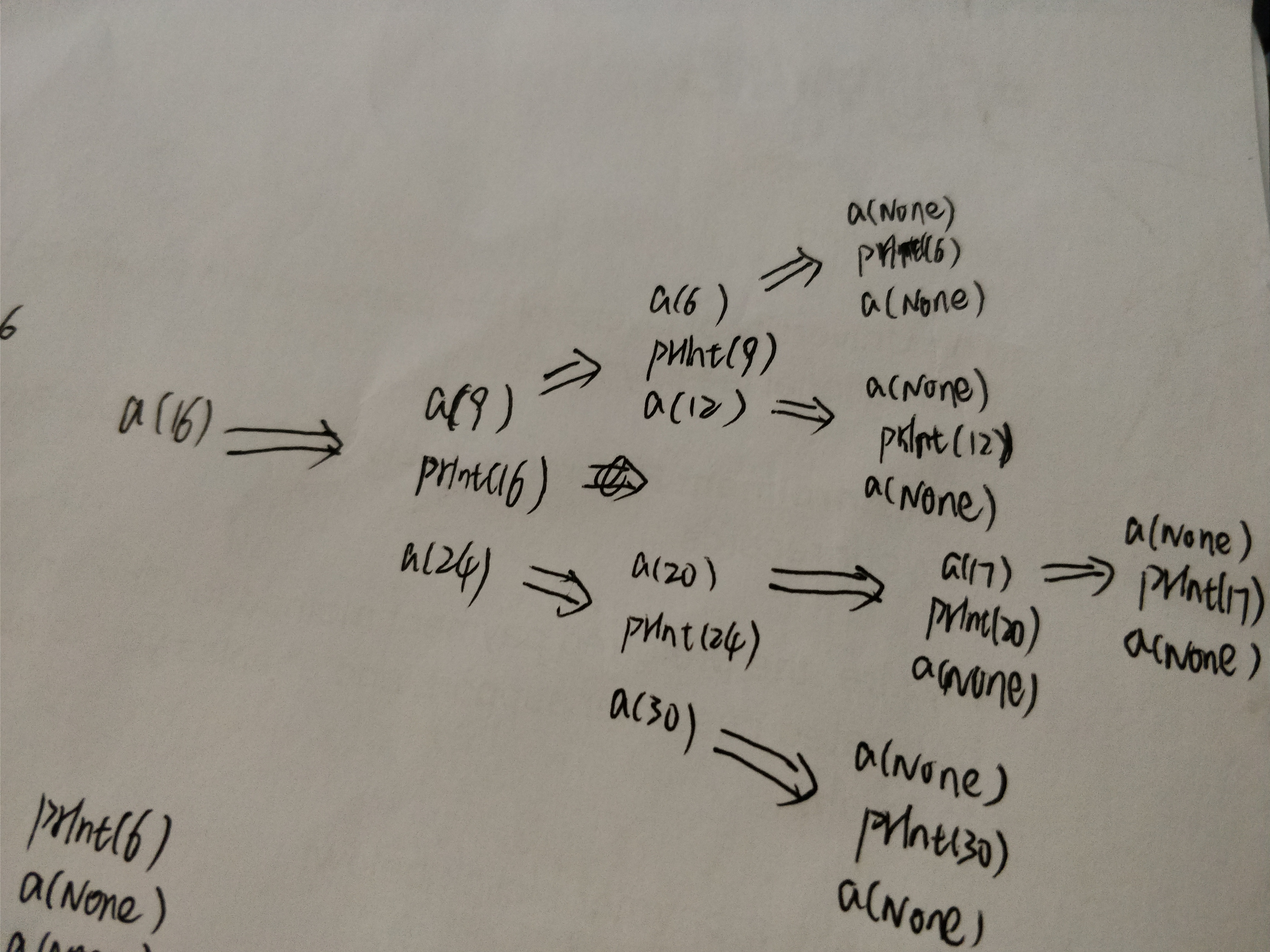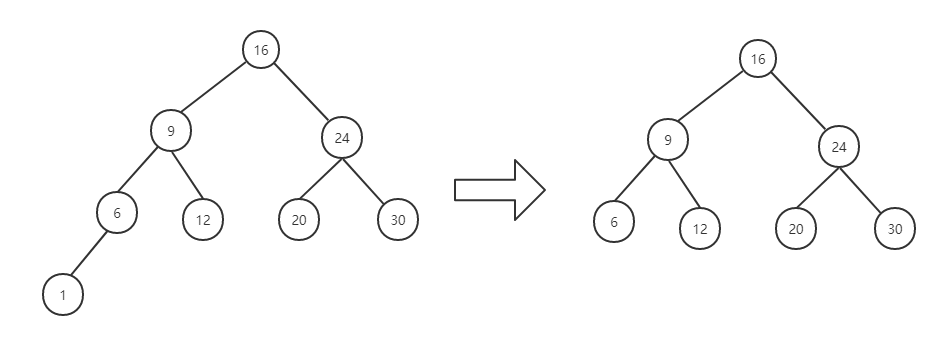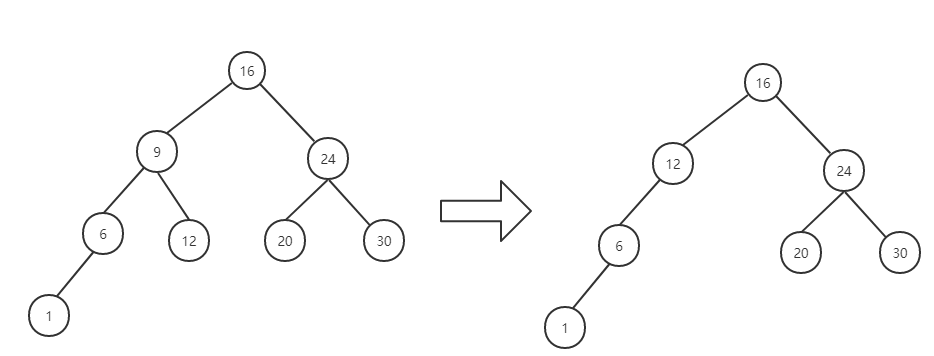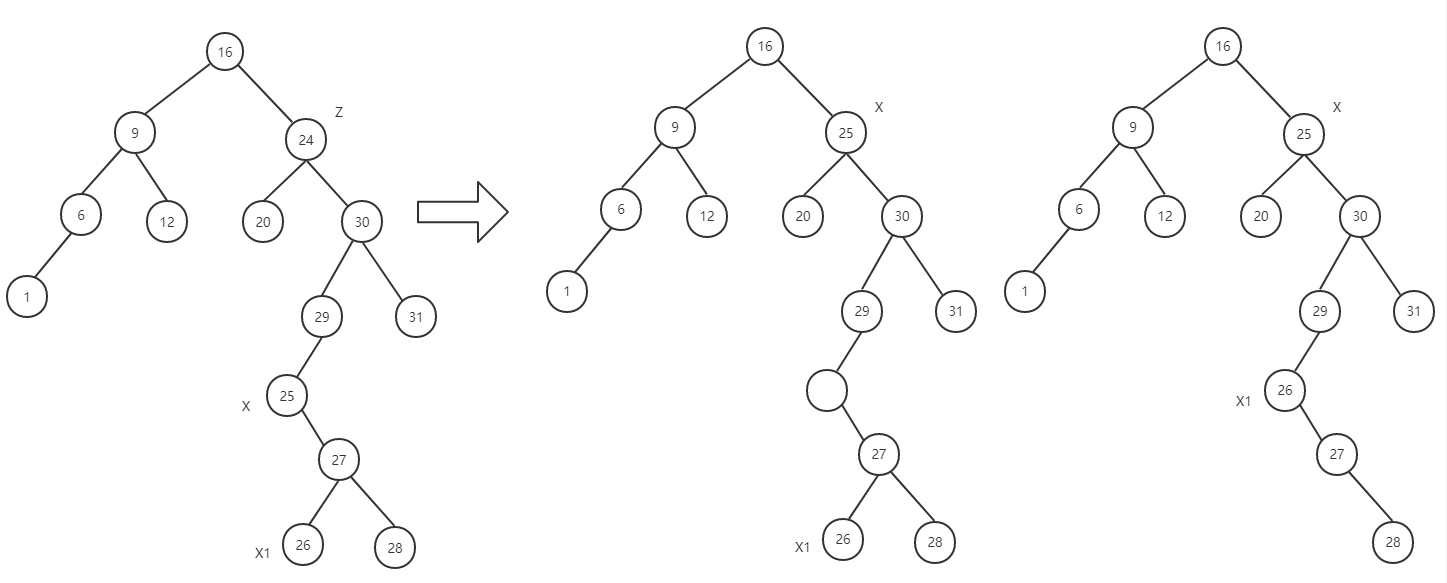# Python 实现二叉搜索树（BST）

hresh 422## 前言

• 若左子树非空，则左子树上的所有节点的关键字值均小于根节点的关键字值。
• 若右子树非空，则右子树上的所有节点的关键字值均大于根节点的关键字值。
• 左、右子树本身也分别是一棵二叉查找树（二叉排序树）。## 实战分析

class TreeNode():
def __init__(self, x=None):
self.val = x
self.left = None
self.right = None
self.parent = None

def __str__(self):
return str(self.val)


class BST():
def __init__(self, node=None):
'''
设置根节点
:param node: 根节点
'''
node = TreeNode(node)
self.root_node = node

def insert(self, x):
'''
二叉树做插入操作
:param x:
:return:
'''
if self.is_exist(x):#判断树中是否存在该节点
return
p = TreeNode(x)
if self.root_node == None:#如果树为空的话，则将插入的节点置为根节点
self.root_node = p
else:
cur = self.root_node
pre = None
while cur != None:#利用非递归的方法遍历
pre = cur
if cur.val < x:
cur = cur.right
else:
cur = cur.left

p.parent = pre
if pre.val < x:
pre.right = p
else:
pre.left = p

def is_exist(self, k):
'''
判断二叉树中是否存在某节点的数值与 k 相等
:param k:
:return:
'''
cur = self.root_node
while cur != None and cur.val != k:
if k < cur.val:
cur = cur.left
else:
cur = cur.right
return True if cur != None else False

if __name__ == '__main__':
bs_tree = BST(16)   #初始化二叉树
#对二叉树做插入操作
bs_tree.insert(9)
bs_tree.insert(24)
bs_tree.insert(12)
bs_tree.insert(6)
bs_tree.insert(20)
bs_tree.insert(30)


1.先序遍历

    def preorder_tree_walk(self, n):
'''
先序遍历树节点（根左右）
:param n:
:return:
'''
if n != None:
print(n.val, end=' ')
self.preorder_tree_walk(n.left)
self.preorder_tree_walk(n.right)


2.中序遍历

    def inorder_tree_walk(self, n):
'''
中序遍历树节点（左根右）,输出结果正好是排序好的顺序
:param n:
:return:
'''
if n != None:
self.inorder_tree_walk(n.left)
print(n.val, end=" ")
self.inorder_tree_walk(n.right)3.后序遍历

    def postorder_tree_walk(self, n):
'''
后序遍历树节点（左右根）
:param n:
:return:
'''
if n != None:
self.postorder_tree_walk(n.left)
self.postorder_tree_walk(n.right)
print(n.val, end=' ')


1.查找

    def find_node(self, k):
'''
找到值为k的节点并返回
:param k:
:return:
'''
cur = self.root_node
while cur != None and cur.val != k:
if k < cur.val:
cur = cur.left
else:
cur = cur.right
return cur


BST 的查找是从根节点开始，若二叉树非空，将给定值与根节点的关键字比较，若相等，则查找成功；若不等，则当给定值小于根节点关键字时，在根节点的左子树中查找，否则在根节点的右子树中查找。采用非递归的方式求解。

2.查找第 k 大值

    def search(self, k):
'''
二叉搜索树按照中序遍历的顺序打印出来正好就是排序好的顺序
从二叉树中查找第k大的节点元素
:param k:
:return:
'''
count = 0
val = None
flag = False

def inorder(n):
nonlocal count,val,flag

# if flag:
#     return

if n != None:
inorder(n.left)
count += 1
print(n.val, count,k,flag)
if count == k:
val = n.val
flag = True
n.left = None
n.right = None
if count > k:
return

inorder(n.right)

inorder(self.root_node)
return val


3.节点左子树最大值

    def find_max_node(self, node):
'''
查找node节点左子树中最大的那个节点，按照中序遍历（左根右）的顺序可知，左子树最大的那个节点通过判断node.right不断往下查找
:param node:
:return:
'''
np = TreeNode()
np = node

if np == None:
return None
while np.right != None:
np = np.right
return np


4.节点右子树最小值

    def find_min_node(self, node):
'''
查找node右子树上最小的节点
:param node:
:return:
'''
np = TreeNode()
np = node

if np == None:
return None
while np.left != None:
np = np.left
return np


• 若被删除节点 z 是叶子节点，则直接删除，修改 z 的父节点的指向，不会破坏二叉搜索树的性质；
• 若节点 z 的后继 x （如果有右子树，则提取的是右子树中的最小值，否则提取的是左子树中的最大值）， z 是 x 的父节点，则让 x 代替 z 的位置；
• 若节点 z 的后继 x （如果有右子树，则提取的是右子树中的最小值，否则提取的是左子树中的最大值）， z 与 x 不直接相连，则先删除节点 z，把 x 放在 z 的位置，同样还要考虑 x 的后继 x1 ，这样递归下去，直到转换成第一或第二种情况。def delete_leaf(self, node):
cur_parent = node.parent
if cur_parent != None:
if node.val < cur_parent.val:
cur_parent.left = None
else:
cur_parent.right = None
else:
self.root_node = None

def delete(self, node):

if self.is_leaf(node):#为叶子节点时，直接删除
self.delete_leaf(node)
else:
replace_node = self.find_min_node(node.right) if node.right != None else self.find_max_node(node.left)
# replace_node = self.find_max_node(node.left) if node.left != None else self.find_min_node(node.right)

rep_node = TreeNode()
rep_node = copy.copy(replace_node)#浅复制，保持原有的节点指向

if node == replace_node.parent:#如果后继和node相关联，则按照以下方法处理
if node.val < node.parent.val:
node.parent.left = replace_node
else:
node.parent.right = replace_node
replace_node.parent = node.parent
if replace_node != node.left:
replace_node.left = node.left
node.left.parent = replace_node
else:#删除 node 节点后，更新后继节点的父节点和左节点和右节点
nd_parent = node.parent

if nd_parent != None:
if node.val > nd_parent.val:
nd_parent.right = replace_node
else:
nd_parent.left = replace_node
else:
self.root_node = replace_node
replace_node.parent = nd_parent

replace_node.left = node.left
replace_node.right = node.right

if node.left != None:
node.left.parent = replace_node
if node.right != None:
node.right.parent = replace_node
# print(rep_node)

self.delete(rep_node)